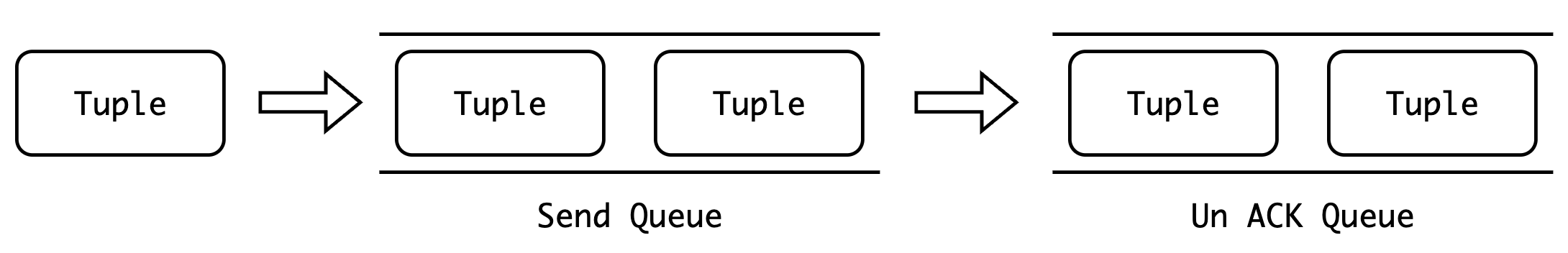Greenplum Squelch 机制用于提前终止执行节点的执行，和 PostgreSQL 单机节点不同的是，Greenplum 无法通过停止调用函数停止节点的执行，因为 Greenplum 涉及到不同节点之间的数据传输。

### 1. 基于 UDP 的 Greenplum Interconnect 控制流程

Interconnect 是建立的 UDP 协议之上的应用层数据传输协议，在原本不可靠 UDP 之上自行实现了可靠传输、流量控制等功能。那么为什么不直接使用 TCP 协议，而是大费周章地再去实现一个可靠的 UDP 协议呢？原因在于 TCP 协议是点对点的有状态传输协议，如果我们 N 个节点执行 RedistributeMotion 的话，那么就会产生 \$N^2\$ 个连接。假如当前集群有 500 个 segment 的话，那么一个 RedistributeMotion 就会有 500*500 = 250,000 个 TCP 连接，一次性建立这么多个连接是相当低效的。1. 序列化 Tuple，将其组装为数据包，并添加至发送队列中
2. 遍历发送队列，发送数据包，并把数据包从发送队列中删除，插入到未确认队列中
3. 读取接收端的 ACK 数据包，从未确认队列中删除已收到 ACK 的数据包

### 3. 历史中关于 Squelch 机制的 BUG

#### 1. 提前终止 SharedInputScan 节点导致 Hang 住

``````CREATE TABLE foo (a int, b int);
CREATE TABLE bar (c int, d int);
CREATE TABLE jazz(e int, f int);

INSERT INTO bar  VALUES (1, 1), (2, 2), (3, 3);
INSERT INTO jazz VALUES (2, 2), (3, 3);

ANALYZE foo;
ANALYZE bar;
ANALYZE jazz;

EXPLAIN SELECT * FROM
(
WITH cte AS (SELECT * FROM foo)
SELECT * FROM (SELECT * FROM cte UNION ALL SELECT * FROM cte)
AS X JOIN bar ON b = c
) AS XY JOIN jazz on c = e AND b = f;
``````

``````                                                 QUERY PLAN
------------------------------------------------------------------------------------------------------------
Gather Motion 3:1  (slice2; segments: 3)  (cost=0.00..2155.00 rows=1 width=24)
->  Hash Join  (cost=0.00..2155.00 rows=1 width=24)
Hash Cond: bar.c = jazz.e AND share0_ref2.b = jazz.f AND share0_ref2.b = jazz.e AND bar.c = jazz.f
->  Sequence  (cost=0.00..1724.00 rows=1 width=16)
->  Shared Scan (share slice:id 2:0)  (cost=0.00..431.00 rows=1 width=1)
->  Materialize  (cost=0.00..431.00 rows=1 width=1)
->  Table Scan on foo  (cost=0.00..431.00 rows=1 width=8)
->  Hash Join  (cost=0.00..1293.00 rows=1 width=16)
Hash Cond: share0_ref2.b = bar.c
->  Redistribute Motion 3:3  (slice1; segments: 3)  (cost=0.00..862.00 rows=1 width=8)
Hash Key: share0_ref2.b
->  Append  (cost=0.00..862.00 rows=1 width=8)
->  Shared Scan (share slice:id 1:0)  (cost=0.00..431.00 rows=1 width=8)
->  Shared Scan (share slice:id 1:0)  (cost=0.00..431.00 rows=1 width=8)
->  Hash  (cost=431.00..431.00 rows=1 width=8)
->  Table Scan on bar  (cost=0.00..431.00 rows=1 width=8)
->  Hash  (cost=431.00..431.00 rows=1 width=8)
->  Table Scan on jazz  (cost=0.00..431.00 rows=1 width=8)
Filter: e = f
Optimizer status: PQO version 2.39.1
(20 rows)
````````````switch (share_type)
{
case SHARE_MATERIAL:
case SHARE_SORT:
return CdbVisit_Skip;

case SHARE_MATERIAL_XSLICE:
case SHARE_SORT_XSLICE:
if (isWriter && !tuplestoreInitialized)
ExecProcNode(node);     /* 继续执行该节点，而不是取消 */
break;
case SHARE_NOTSHARED:
break;
}
``````

#### 2. 嵌套的 SharedInputScan

``````create table t1(a int, b int);
create table t2(a int, b int);
create table t3(a int, b int);
create table t4(a int, b int);
create table t5(a int, b int);

insert into t1 select i,i from generate_series(1, 10000)i;
insert into t2 select i,i from generate_series(1, 10000)i;

analyze;

explain (costs off) select from
(
with cte1(a,b) as (select t1.a, t2.a from t1, t2 where t1.a = t2.b),
cte2(a,b) as (select xx.a+2, xx.b-5 from cte1 xx, cte1 yy where xx.a = yy.a)
select xx.a, count(1) from cte2 xx, cte2 yy where xx.a = yy.a group by xx.a union all select 1, 2 from cte1 x, cte2 y where x.a > y.b
) x (a, b)  join  t3
on  x.a = t3.a;
``````

``````                                             QUERY PLAN
-----------------------------------------------------------------------------------------------------
Gather Motion 3:1  (slice6; segments: 3)
->  Hash Join
Hash Cond: (share1_ref3."?column?" = t3.a)
->  Sequence
->  Shared Scan (share slice:id 6:0)
->  Materialize
->  Hash Join
Hash Cond: (t2.b = t1.a)
->  Redistribute Motion 3:3  (slice4; segments: 3)
Hash Key: t2.b
->  Seq Scan on t2
->  Hash
->  Seq Scan on t1
->  Sequence
->  Shared Scan (share slice:id 6:1)
->  Materialize
->  Result
->  Hash Join
Hash Cond: (share0_ref3.a = share0_ref2.a)
->  Shared Scan (share slice:id 6:0)
->  Hash
->  Shared Scan (share slice:id 6:0)
->  Append
->  HashAggregate
Group Key: share1_ref3."?column?"
->  Hash Join
Hash Cond: (share1_ref3."?column?" = share1_ref2."?column?")
->  Redistribute Motion 3:3  (slice1; segments: 3)
Hash Key: share1_ref3."?column?"
->  Result
->  Shared Scan (share slice:id 1:1)
->  Hash
->  Redistribute Motion 3:3  (slice2; segments: 3)
Hash Key: share1_ref2."?column?"
->  Result
->  Shared Scan (share slice:id 2:1)
->  Result
->  Result
->  Nested Loop
Join Filter: (share0_ref4.a > share1_ref4."?column?_1")
->  Shared Scan (share slice:id 6:1)
->  Materialize
->  Broadcast Motion 3:3  (slice3; segments: 3)
->  Result
->  Shared Scan (share slice:id 3:0)
->  Hash
->  Broadcast Motion 3:3  (slice5; segments: 3)
->  Seq Scan on t3
Optimizer: Pivotal Optimizer (GPORCA)
``````

``````ERROR:  cannot execute squelched plan node of type: 232 (execProcnode.c:947)  (seg0 slice6 127.0.1.1:6002 pid=92140) (execProcnode.c:947)
``````